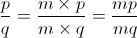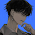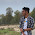## Saturday, 8 December 2012

### CBSE Class 7 - Maths - CH8 - Comparing Quantities (Ratio and NCERT Ex 8.1)

Comparing Quantities(Ratio)Ratio of Circumference: Diameter = π image credits: openclipart

Important Points

1. In the ratio x:y (called as x is to y), x is called the first term or antecedent. y is called the second term or consequent.

2. While comparing two quantities, the units must be the same.

3. A ratio remains unchanged if both its terms are multiplied or divided by same non-zero number.4. The ratio (p:q) is said to be in simplest form when HCF of p and q is 1.

NCERT Exercise 8.1

Q1: Find the ratio of:
(a) ₹ 5 to 50 paise
(b) 15 kg to 210 g
(c) 9 m to 27 cm
(d) 30 days to 36 hours

(a) ₹ 5 to 50 paise
Since units are not same, we need to convert both to same units.
₹ 1 = 100 paise     ∴ ₹ 5 = 5 × 100 = 500 paise
∴ ₹ 5 to 50 paise = 500 paise: 50 paise = 500/50 = 10:1

(b) 15 kg to 210 g
Since units are not same, we need to convert both to same units.
∵ 1 kg  = 1000g   ∴ 15kg = 15 × 1000 = 15000g
∴ 15 kg to 210 g = 15000g : 210g = 15000/210 = 500:7

(c) 9 m to 27 cm
Converting both terms to same units.
∵ 1m = 100 cm   ∴ 9m = 9 × 100cm = 900cm
∴ 9m to 27cm = 900cm : 27cm = 900 / 27 = 100/3 = 100:3

(d)  30 days to 36 hours
Converting both terms to same units.
∵ 1 day = 24 hours
∴ 30 days = 30 × 24 hours = 720 hours
∴ 30 days to 36 hours = 720 hours : 36 hours =720/36 = 20/1 = 20:1

Q2: In a computer lab, there are 3 computers for every 6 students. How many computers will be needed for 24 students?Q3:Population of Rajasthan = 570 lakhs and population of UP = 1660 lakhs.
Area of Rajasthan = 3 lakh km2 and area of UP = 2 lakh km2.
(i) How many people are there per km2 in both these States?
(ii) Which State is less populated?

Let us calculate ratio as population per area.
Population of Rajasthan per 3 lakh km2 = 570 lakh
Population of Rajasthan per 1 lakh km2 =570/3 = 190 lakh      ... (I)

Population of UP per 2 lakh km2 = 1660 lakh
Population of UP per 1 lakh km2 = 1660/2 = 830 lakh             ...(II)

Comparing values I and II, we can say population ratio per area of  Rajasthan is less.

Other Questions on Ratio

Q4: Express 24:30 in its simplest form.

Answer: Ratio in simplest form is when HCF of two terms is 1 i.e. there is no common factor except one.

∴ 24 : 30 = 4 × 2 × 3 : 5 × 2 × 3 = 4 : 5

Q5: Express ratio 1m 5cm : 63 cm in simplest form.

Answer: Converting into same units.
∵ 1m = 100 cm
∴ 1m 5cm = 100 cm + 5 cm = 105 cm
∴ 1m 5cm : 63 cm = 105 cm : 63 cm = 7 × 5 × 3 : 7 × 3 × 3 = 5 : 3

Q6: Divide ₹ 360 in the ratio 7:8.

Answer:  Let the two values after division is 7x and 8x.
Sum of these values = 7x + 8x = 15x = ₹ 360
⇒ x = 360/15 = 24

∴ First value = 7x = 7 × 24 = ₹ 168
and the second value = 8x = 8 × 24 = ₹ 192

Thus  ₹360 is divided into ₹168 and ₹192 as per the given ratio (7:8)

Q7: Two numbers are in the ratio of 7:11. If 7 is added to each of the numbers the ratio becomes 2:3. Find the numbers.

Let the first number = 7p
and the second number = 11p

After adding 7, the first number becomes = 7p + 7
After adding 7, the second number becomes = 11p + 7

The new ratio is,

Thus numbers are 49 and 77.

Q8: If 15 oranges cost Rs 70, what do 39 oranges cost?

Answer: Cost of 15 oranges = ₹70
Cost of 1 orange     = 70/15
Cost of 39 oranges = 70 × 39 /15 = 14 × 13 = ₹182

Q9: Fill in the blanks:
1. The comparison of two quantities of the same kind by means of division is termed as_____________.
2. The two quantities to be compared are called the ________ of the ratio.
3. The first term of the ratio is called the _________ and the second term is called the _______.
4. Equality of two ratios is called a __________. If a,b;c,d are in proportion,then a:b::c:d .
5. In a proportion, the product of extremes =___________
1. ratio
2. terms
3. antecedent, consequent
4. proportion
5. product of means
.1.2.thanks really use ful

3.4.Thanks for helping me

5.Thanks for helping me

6.Its useful 🤓🤓🙄😊

7.Good material for practice

8.not useful